# MODI Method Examples: Transportation Problem

In the previous section, we provided the steps in MODI method (modified distribution method) to solve a transportation problem.

In this section, we provide an example. Let's solve the following example:

This example is the largest and the most involved you have read so far. So you must read the steps and the explanation mindfully.

##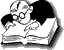Example: MODI Method (Modified Distribution Method)

Consider the transportation problem presented in the following table.

On small screens, use horizontal scrollbar to view full table

Distribution centre
D1 D2 D3 D4 Supply
Plant P1 19 30 50 12 7
P2 70 30 40 60 10
P3 40 10 60 20 18
Requirement   5 8 7 15

Determine the optimal solution of the above problem.

Solution.

An initial basic feasible solution is obtained by Matrix Minimum Method and is shown in table 1.

Table 1

Distribution centre
D1 D2 D3 D4 Supply
Plant P1 19 30 50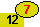7
P2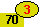30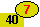60 10
P3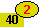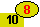60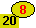18
Requirement   5 8 7 15
##### Initial basic feasible solution

12 X 7 + 70 X 3 + 40 X 7 + 40 X 2 + 10 X 8 + 20 X 8 = Rs. 894.

Calculating ui and vj using ui + vj = cij

Substituting u1 = 0, we get

u1 + v4 = c14 ⇒ 0 + v4 = 12 or v4 = 12
u3 + v4 = c34 ⇒ u3 + 12 = 20 or u3 = 8
u3 + v2 = c32 ⇒ 8 + v2 = 10 or v2 = 2
u3 + v1 = c31 ⇒ 8 + v1 = 40 or v1 = 32
u2 + v1 = c21 ⇒ u2 + 32 = 70 or u2 = 38
u2 + v3 = c23 ⇒ 38 + v3 = 40 or v3 = 2

Table 2

Distribution centre
D1 D2 D3 D4 Supply ui
Plant P1 19 30 507 0
P23060 10 38
P36018 8
Requirement   5 8 7 15
vj   32 2 2 12

Calculating opportunity cost using cij – ( ui + vj )

Unoccupied cells Opportunity cost
(P1, D1) c11 – ( u1 + v1 ) = 19 – (0 + 32) = –13
(P1, D2) c12 – ( u1 + v2 ) = 30 – (0 + 2) = 28
(P1, D3) c13 – ( u1 + v3 ) = 50 – (0 + 2) = 48
(P2, D2) c22 – ( u2 + v2 ) = 30 – (38 + 2) = –10
(P2, D4) c14 – ( u2 + v4 ) = 60 – (38 + 12) = 10
(P3, D3) c33 – ( u3 + v3 ) = 60 – (8 + 2) = 50

Table 3

Use Horizontal Scrollbar to View Full Table Calculation

Distribution centre
D1 D2 D3 D4 Supply ui
Plant P1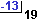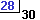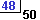7 0
P2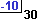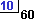10 38
P3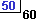18 8
Requirement   5 8 7 15
vj   32 2 2 12

Now choose the smallest (most) negative value from opportunity cost (i.e., –13) and draw a closed path from P1D1. The following table shows the closed path.

Table 4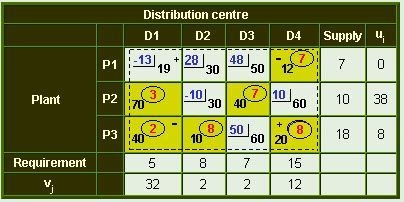Choose the smallest value with a negative position on the closed path(i.e., 2), it indicates the number of units that can be shipped to the entering cell. Now add this quantity to all the cells on the corner points of the closed path marked with plus signs and subtract it from those cells marked with minus signs. In this way, an unoccupied cell becomes an occupied cell.

Now again calculate the values for ui & vj and opportunity cost. The resulting matrix is shown below.

Table 5

Distribution centre
D1 D2 D3 D4 Supply ui
Plant P1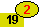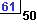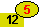7 0
P2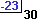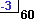10 51
P3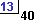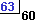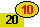18 8
Requirement   5 8 7 15
vj   19 2 –11 12

Choose the smallest (most) negative value from opportunity cost (i.e., –23). Now draw a closed path from P2D2 .

Table 6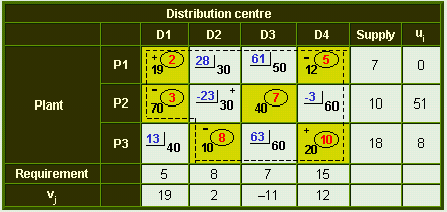Now again calculate the values for ui & vj and opportunity cost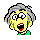Don't panic. The following table is the last table.

"Every complex human problem has an easy solution - neat & small, but wrong." - Vinay Chhabra & Manish Dewan

### Table 7: MODI Method

Distribution centre
D1 D2 D3 D4 Supply ui
Plant P1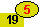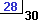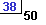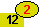7 0
P2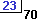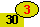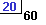10 28
P3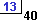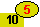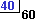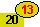18 8
Requirement   5 8 7 15
vj   19 2 12 12

Since all the current opportunity costs are non–negative, this is the optimal solution. The minimum transportation cost is: 19 X 5 + 12 X 2 + 30 X 3 + 40 X 7 + 10 X 5 + 20 X 13 = Rs. 799

•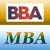MBA & BBA
•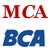BCA & MCA
•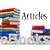Ebooks & Articles
•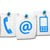Contact us